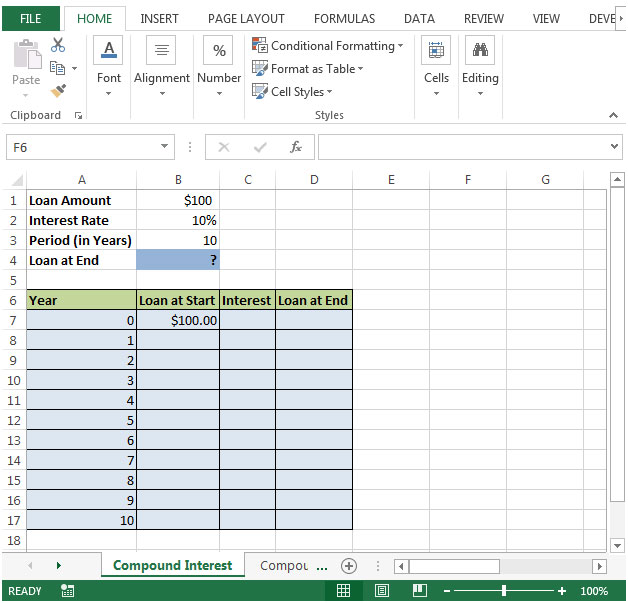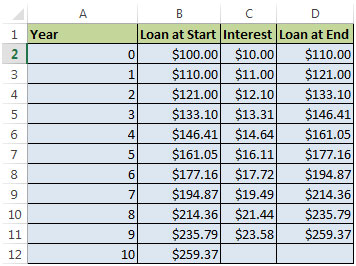# How to create Compound Interest calculator in Microsoft Excel

What is Compound Interest?

Compound interest is the formula to calculate the interest of loan. In this article, we will learn the formula that can be used to calculate the quarterly compound rate of interest in Microsoft Excel.

&nbsp
Let us take an example to calculate compound interest:-

If loan amount of \$100 is provided by Bank at an Interest Rate of 10%, then what will be theloan amount at the end of 10 years?With Compound Interest, we can work out the interest for the first year, add it to the totaland then calculate the interest for the next year and continue the same till the given time (in years).

Here are the calculations for a 10 Year Loan at 10%:Let us see how we calculate Loan at End step by step:

• Calculation of the Interest (=Loan at Start x Rate of Interest)
• Add the amount of Interest to the ‘Loan at Start’ to get the ‘Loan at End’ of the year
• Loan at start date is \$100 & after 10 years, customer has to pay \$259.37
• This means customer is paying \$159.37 extra at 10 % Interest Rate which is more than double of the loan amount

There are more simple ways to calculate the ‘Loan at End’

• Loan at Start + (Loan at Start x Interest)
• \$100 + (\$100 x 10%) = \$ 110

Adding 10% interest is the same thing as we are multiplying by 1.10

The basic concept of calculating compound interest is that interest is added back to the principal sum so that interest is earned on that added interest during the next compounding period.
&nbsp